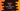# Python program to find the sum of all numbers in a file## Python program to find the sum of all numbers in a file:

In this python tutorial, we will learn how to find the sum of all numbers found in a file. With this program, you will learn basic file handling in python like how to read the contents of a file and how to check each character one by one.

### Algorithm to find the sum:

Below algorithm we will use to find the sum of all numbers in a file:

• The file path is given. Open the file in read mode.
• Initialize one variable to hold the sum of all numbers we will find in the file. Initialize it as 0.
• Read all contents of the file as lines.
• Iterate through the lines one by one.
• For each line, iterate through the characters one by one.
• For each character, check if the character is a digit or not, if yes, add it to the sum variable. Else, ignore it.
• Once the iteration is completed, print out the sum to the user.

### Python program:

Below is the complete python program:

``````given_file = open('input.txt', 'r')

sum = 0

for line in lines:
for c in line:
if c.isdigit() == True:
sum = sum + int(c)

print(sum)

given_file.close()``````

Here,

• input.txt is the file path. To run this program, you need to create one input.txt file in the same folder. If you want to run it for a different file, you can put the file path in place of input.txt.
• open is used to open a file in python. We are passing r as the second parameter. It indicates that we are opening the file in read mode. We are not doing any write operation on the file. So, opening it in write mode is enough.
• readlines method returns all lines of a file. We are storing that value in the lines variable.
• sum is used to hold the sum of all digits in the file. It is initialized as 0.
• The first line of the for loop is used to iterate through all lines one by one.
• For each line, we are iterating through the characters one by one.
• isdigit is used to check if a character is a digit or not. If it returns True, i.e. if it is a digit, we are adding that value to sum.
• Finally, after the loops are ended, we are printing the value of sum to the user.

### Sample output:

For example, if we have input.txt file with the below content:

``````hello1
world 23       4
new line    5
new line one more 6

7``````

If we run the above program, it will print 28 as the output.